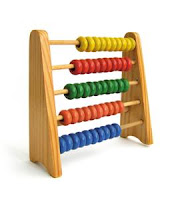# LCM and HCF Shortcuts

• Factor : A number is said to be a factor of other when it EXACTLY divides the other.
• Ex : 6 and 7 are Factors of 42.
• Multiple : A number is said to be a multiple of another, when it is Exactly divisible by the other
• Ex : 42 is a multiple of 6 and 7
Please re - read these definitions. So that you can get the difference between Factor and Multiple.• Prime Number : Prime number is a number which has no factors except itself and Unity.
• Ex  :2, 3, 5, 7, 11, 13, 17 etc are prime numbers
• Composite Number :  Composite number is a number which has other factors besides itself and Unity.
• Ex : 14, 15, 16, 18 etc
• Co-Prime : Two numbers are said to be Co-Prime (Prime-To-Each Other) when they have no common factors except Unity.
• Note : The Co-Primes need not necessarily be Primes.
• 15 and 19
• 15, 17 and 22          are        Co-Primes
• Common Multiple : A Common Multiple of two or more numbers is a number which is exactly divisible by each of them.
• Ex : 12 is a common multiple of 2, 3, 4 and 6
• Least Common Multiple  (LCM) : The LCM of two or more given numbers is the Least Number which is exactly divisible by each of them.
• Ex  :
• 20 is the Common Multiple of 2, 4, 5 and 10
• 40 is the Common Multiple of 2, 4, 5 and 10
• 80 is the Common Multiple of 2, 4, 5 and 10,          But
• Here 20 is the Least Common Multiple of 2, 4, 5, and 10
• Highest Common Factor (HCF) : The HCF of two or more numbers is the Greatest Number which divides each of them Exactly.
• It is also Called Greatest Common Divisor (GCD)
• Ex : Find the HCF of 18, 24
• Factors of 18 --> 1, 2, 3, 6, 9, 18
• Factors of 24 --> 1, 2, 3, 4, 6, 8, 12, 24
• Here the Greatest number, which divides them exactly is 6. So 6 is the H.C.F of 18, 24
In the above example they have given very small numbers. So it was easy for us to find the HCF. What if they ask you to find the HCF for 84 and 540 ? Will you write the factors to both of them and then find out the Highest number? If you are planning to do that , please erase that thought from your mind :) Because there are several methods to make the process simple

Methods of finding HCF :
• HCF by factorization :
1. Express each of the given number as the product of Prime Factors
2. Choose common factors
3. Find the Product of Lowest Power of these Factors.
• This Product  is the required HCF of the given Numbers
Ex : Find the HCF of 84, 540
If you find this method lil confusing, dont worry. There is another method to find HCF.

• HCF by Method of Division :
• Consider two different numbers.
• Divide the longer number by the smaller one.
• Now divide the divisor by the reminder.
• Repeat this process of dividing the preceding divisor by the last reminder obtained, till you get the reminder "0"
• The LAST DIVISOR is the HCF of the given TWO numbers
Ex : Find the HCF of 42, 70

Thats it. Now the answer is 14 :)

1.i hav doubt in lcm ,u said 40 cmn mul r 2, 4, 5, 10 y v shouldn't include 8 also , pls explain . .

1.Well it seems you dint get the concept...

See, here we are talking about the COMMON MULTIPLES of the given numbers.

Assume that you've taken 8 too into consideration...

80 is the common multiple of 2, 4, 5, 8 and 10
40 is the common multiple of 2, 4, 5, 8 and 10
but what about 20? Its not the multiple of 8 no? That's the reason why we dint took 8 into consideration for this example :)

2.ya sir i got it now , thanks for ur kind reply

3.Hello sir in the above answer u said didn't took so is day a right one while writing r speaking I'm. Getting confusion pls explain me sir

4.I'm a Maths teacher Naresh... Usually maths teachers don't have good English speaking skills... That't the reason why dint took :D
(well, sorry for the mistake.. I was in a hurry while responding to that message and you should use TAKE)
Thank you so much for the correction. Good day.....

5.u had given wrong examples for composite number's ma'am. they are 4,6,8,9,...but not 14,16,18....this minor mistakes makes me to get cmpletely confused for a while.

1.You are still in confusion Srinu Rao.. see

14 is a composite number because it has other factors besides itself and unity (2x7, 7x2)
16 is a composite number because it has other factors besides itself and unity (2x8, 4x4, 2x8)
18 is a composite number because it has other factors besides itself and unity (2x9, 3x6, 6x3, 9x2)

hope you got it now :)

6.Very impressive

7.mind blowing yar.. :)

8.Hi how to find LCM of 1.20,30,40 2.10,15

9.kindly explain HCF

10.kindly how to find LCM for 52,46,58 clear explain

11.Shiv RK-u r a nice person...

12.I just want to know some short cut tricks on maths

13.I am a grt fan of u-Shiv RK...